Fatigue Index

Fatigue High Cycles Finite Life

 Fatigue High Cycles Finite Life For many applications the number of stress cycles experienced by a component during its service life is between 10 3 and 10 6 cycles.  For these applications it is economical to use appropriate fatigue strength as opposed to using the endurance limit.  The strength value between the low cycle stress value S'l and the endurance limit value S'e can be plotted on a graph of log10 Fatigue strength (S) vs log10 Number of cycle (N't) as a straight line following the equation.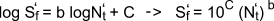b = the slope of the line C = the intercept of the line with the vertical cycles ordinate N't = Number of cycles S'f = the fatigue strength value S'e = the endurance limit at 106 cycles S'l = the fatigue strength at 103 cycles At the endpoints of the high cycles finite life rang (103)cycles and (106)cycles ... The endurance strength values are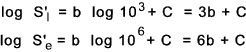subtracting the equation for S'e from S 'l results is the following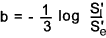To obtain C it is simply necessary to substitute the above into the equation for S'e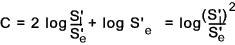Therefore knowing the low level fatigue strength (S'l) for a metal and the endurance strength (S'e) and the number of operating cycles it is possible to obtain the relevant fatigue value using the equation.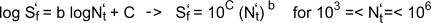If the fatigue strength is known and the number of cycles to failure is needed then the following formula applies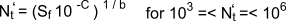Example: to determine the strength at 105 cycles when the strength at 103 and 106 are known.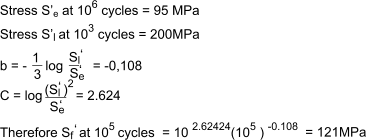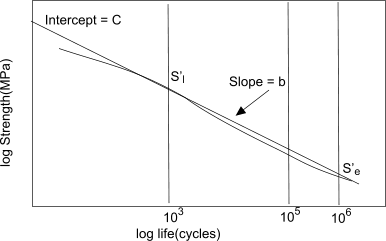Useful Links.. MATWEB ... Vast source of material data including some fatigue values Fatigue Tests on Welded Joints ... Useful informative report download Fatigue Design & Evaluation Committee of the SAE ... Includes a accessible database of fatigue reports on SAE steels Efatigue.com... Excellent Source of Fatigue data. (Based on US materials ) Fatigue Basics -epi_eng ...Useful set of notes
Fatigue Index